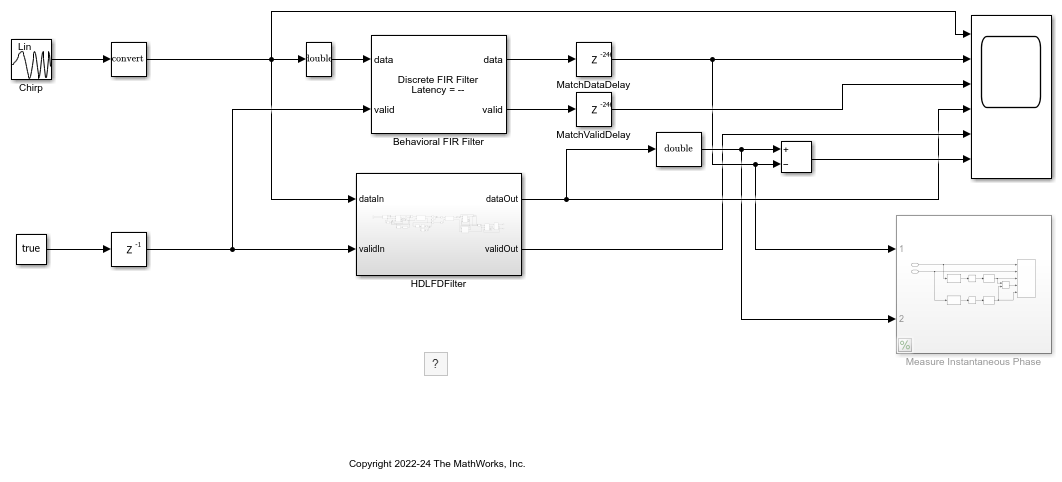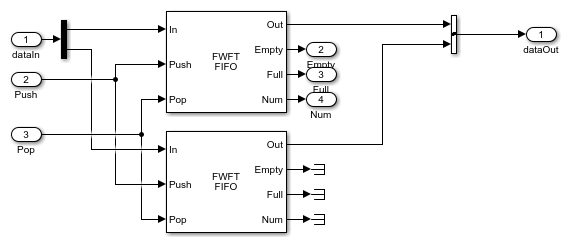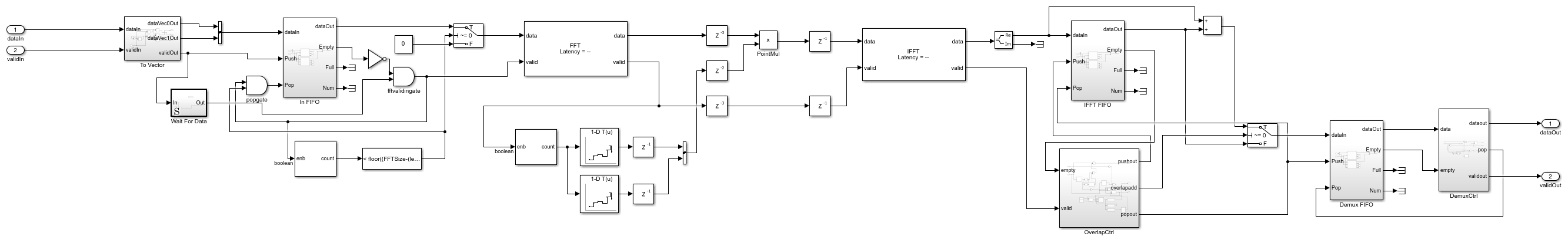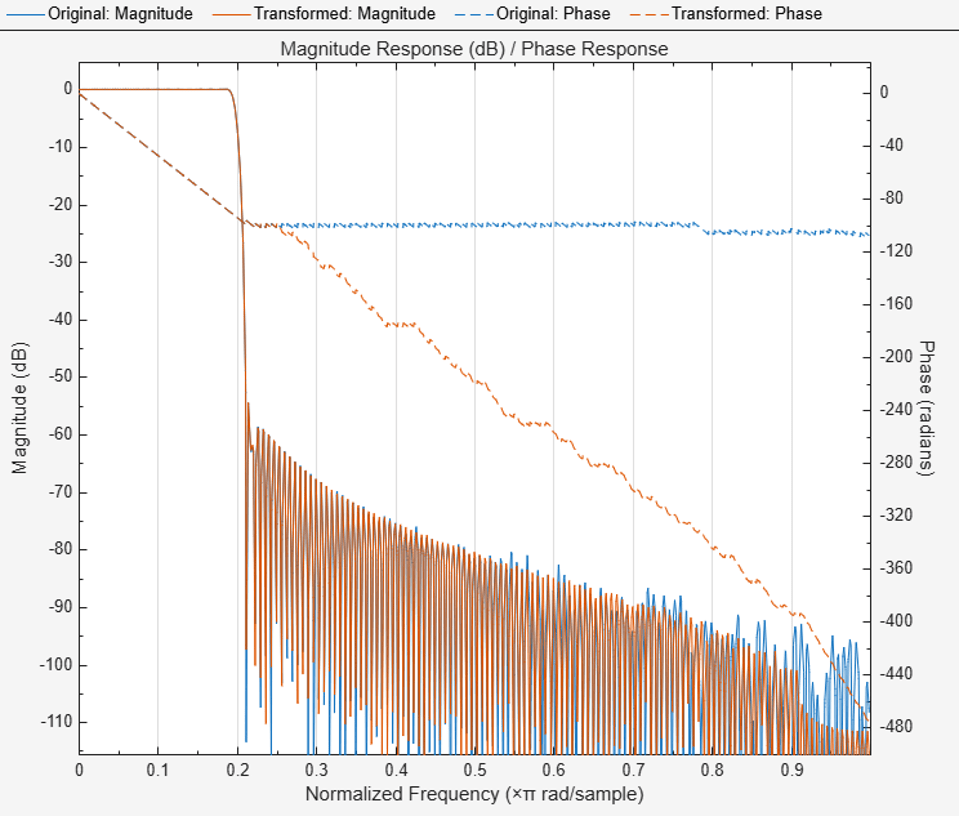# Frequency-Domain Filtering in HDL

This example shows how to implement a filter in the frequency domain.

A sinusoidal input is filtered with the overlap-add method using a frequency-domain FIR filter built using the DSP HDL Toolbox FFT and IFFT blocks. The overlap-add and overlap-save filtering methods are area efficient ways to compute the discrete convolution of a signal with a finite-impulse response (FIR filter). The efficiency gain is particularly noticeable for high-order filters with a large number of taps. Match filters in communications systems, high-order FIR filters, and channel models on FPGA/ASIC hardware are all good examples of systems where frequency-domain filtering is a good choice.

### Open and run the model.

The overlap-add algorithm  filters the input signal in the frequency domain. The input is divided into non-overlapping blocks which are linearly convolved with the FIR filter coefficients. The linear convolution of each block is computed by multiplying the discrete Fourier transforms (DFTs) of the block and the filter coefficients, and computing the inverse DFT of the product. For filter length M and FFT size N, the last M-1 samples of the linear convolution are added to the first M-1 samples of the next input sequence. The first N-M+1 samples of each summation result are output in sequence.

```modelname = 'FreqDomainFiltHDL'; open_system(modelname); set_param(modelname,'SampleTimeColors','on'); set_param(modelname,'Open','on'); set(allchild(0),'Visible','off'); ```### Selecting the FFT Size

In selecting an FFT size for a particular filter, you must take care to make a hardware-friendly choice. If the filter length is an exact power of two, then your FFT size must be exactly double your filter length. In this case with a power of two size, there will be no time, except for the first filter length of samples, where you will not be adding overlapping results. This simplifies the timing of the design since only the starting period must be handled specially.

If your filter length is not an exact power of two, then you must choose an FFT size that is larger than the next power of two from the filter length. In this example, the filter has 300 coefficients, then the next power of two would be 512, but you must choose 1024 for the FFT size. If you try to use the smaller size of 512 and check the timing, you will find that there are three overlapping regions, not two, and the hardware design becomes more complicated and area intensive.

### Converting to Two-samples at a Time

In hardware, computing the FFT of N-(M-1) samples must be done by zero padding the input to the FFT block after the block of samples is sent in. This padding takes time away from processing the input samples, so somehow you must gain the time it takes to pad the input. One way to accomplish this is to buffer sample into a larger frame of say two samples and process these in a frame-based FFT block. Since you also need to buffer samples for a time, a FIFO is a natural buffer to use at the input.

```open_system([modelname '/HDLFDFilter/In FIFO'],'force'); ```### Point Multiplication

The output of the FFT is then multiplied point-by-point, using a pipelined multiplier, with the FFT of the filter coefficients. In this example, the FFT of the filter coefficients is precomputed but for dynamic filter coefficients, but you can add another FFT to compute the coefficient transform. The result of the point multiply is then sent to an IFFT block to be transformed back into the time domain.

```open_system([modelname '/HDLFDFilter'],'force'); ```### Design Considerations

Another important consideration in making the frequency-domain filter hardware-friendly is that the shift from one sample at a time to two (or other power of 2) samples at a time means that the point at which the design switches from the non-overlapped region to the overlapped region must happen on a boundary that is divisible by two (or the selected power of 2) to avoid difficult timing in the design. You would like to switch between the non-overlapped region and the overlapped region on boundary that lines up with the change to a vector of samples. This adds a condition that your filter length must be divisible by your vector size. Also note that odd-order filters (with an even number of taps) will be required.

In this example, the filter transfer function is designed to be a low-pass filter so using a chirp or swept tone input signal allows you to easily visualize the frequency response of the filter directly in Simulink. You must design this filter with a larger than default Density Factor in order for the filter design to converge to a solution. The designed filter has 300 taps and is fully symmetric and so would require 150 multipliers to implement in the conventional way. By using a frequency-domain filtering approach, you are able to reduce this considerably. Care should be taken when comparing multiplier counts since the FIR implementation uses real-only multipliers but the FFT, point-multiply, and IFFT use complex multipliers and therefore require 3 or 4 real multipliers depending on the architecture selected.

### Exploring the Filter Response

You can explore the filter response by looking at the transformed, then quantized, then inverse transformed coefficients versus the original coefficients.

Num is the filter coefficients you calculated and FFTNum is the transform of those coefficients in double precision. You can simulate what the frequency response of the frequency-domain filter will be by quantizing FFTNum and then translating that back into the time domain.

The quantized transformed filter coefficients for the frequency-domain filter require different quantization settings since they can range between above 1 and below -1. Use the fixed-point tools to find the best precision binary point for the data by supplying only the signedness and the word length. Using fvtool for analysis allows you to overlay the data for both the original and the transformed filter coefficients.

```QNum = fi(Num,1,18); QFDNum = ifft(double(fi(FFTNum,1,18))); QFDNum = QFDNum(1:300); h = fvtool(double(QNum),1,double(QFDNum),1); set(h,'OverlayedAnalysis','phase'); ```As you can see in fvtool, the magnitude responses of the two filters match very closely with some minor variations in the stopband up until frequencies very near Nyquist. The phase response matches very closely in the passband but varies significantly in the stopband. The original filter shows some ripples but nearly constant phase in the stopband while the frequency-domain filter shows a quantized phase response that falls away rapidly. The phase response of the stopband is not normally an factor in filter design, but it is good to know that the frequency-domain filter does not match the original here.

### Going further

You can also see the change in stopband phase response by computing the instantaneous phase for each both the behavioral FIR and the frequency-domain filter. To do this you can uncomment the subsystem Measure Instantaneous Phase and re-run the simulation. The instantaneous phase of the real outputs is computed using Analytic Signal block followed by a Complex to Magnitude-Angle and finally an Unwrap block to make the phase continuous.

The DSP System Toolbox block Frequency-Domain FIR Filter has an option to partition the numerator to reduce latency. Using this option, the filter performs overlap-save or overlap-add on each partition, and combines the partial results to form the overall output. The latency is now reduced to the partition length. Using this technique on an HDL frequency-domain filter is also possible but you will likely use more multipliers depending on the size of your filter.

The transformed filter coefficients are computed from the FFT of a real sequence. This produces conjugate symmetric output so the upper bins of the FFT are the same values as the lower bins but reversed and with the sign of the imaginary part changed. This conjugate symmetry could allow you to save half of the storage in the ROM used to store the transformed coefficients since you can map the upper bins to the lower bins in reverse order and conjugate the result. Note that the first bin representing the DC value of the sequence is not used in the reverse upper bins.

You can compare the synthesis results from an FIR filter with the results from the frequency-domain filter. These results can be hard to predict since they depend on the quantization settings used and since the FFT, point-multiply, and IFFT all operated on complex data, each multiplier is either 3 or 4 real multipliers depending on the block settings. Finding the area cross-over point between the two implementations is challenging to predict as well. In this implementation, you used two words at time in order gain the time needed for the overlap regions and allow continuous input, but if your application does not require continuous input, a lower area implementation is possible.

### References

 Overlap-Add Algorithm: Proakis and Manolakis, Digital Signal Processing, 3rd ed, Prentice-Hall, Englewood Cliffs, NJ, 1996, pp. 430 - 433.

 Overlap-Save Algorithm: Oppenheim and Schafer, Discrete-Time Signal Processing, Prentice-Hall, Englewood Cliffs, NJ, 1989, pp. 558 - 560.